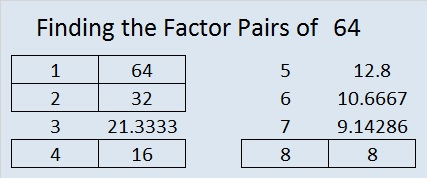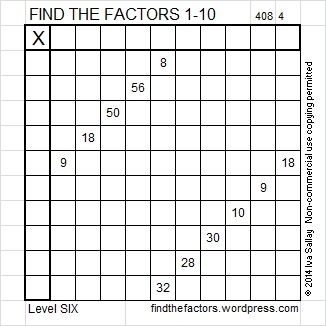# 64 and Can You See the Logic Here?

64 is a composite number, and it is 8 squared. 64 = 1 x 64, 2 x 32, 4 x 16, or 8 x 8. Factors of 64: 1, 2, 4, 8, 16, 32, 64. Prime factorization: 64 = 2 x 2 x 2 x 2 x 2 x 2, which can also be written 64 = 2⁶.Since √64 = 8, a whole number, 64 is a perfect square.

Sometimes 64 is a clue in the FIND THE FACTORS puzzles. Even though it has other factors, when it is a clue, the factors are always 8 x 8.

64 is in this cool pattern:All Previously Published PuzzlesThis solution is complicated, but here is a logical explanation:

There are only two 3s available, so at most one 9 can be 3 x 3.

Can both 9s be 9 x 1? If both were, then both 1s would be used, leaving 8 = 2 x 4 and 10 = 2 x 5 as the only possibilities for 8 and 10. But if both 9s were 9 x 1, then the 18 in the same row as the 9 would have to be 18 = 9 x 2, and that would use too many twos. Therefore, EXACTLY ONE of the nines can use 9 = 9 x 1.

Since one 9 = 3 x 3, using both 3s, 30 must equal 5 x 6. And since both 30 and 50 must use both 5s, 10 must equal 10 x 1.

Since 10 = 10 x 1 and a 9 = 9 x 1, 8 must equal 2 x 4. So now finally we can begin filling out the puzzle:## 4 thoughts on “64 and Can You See the Logic Here?”

1.Joseph Nebus

Got it! … I’m glad this wasn’t one that goes up to the multiples of twelve.

2.ivasallay

You would have found a way to solve it, too.

•ivasallay

Thank you. You are doing exactly what I’ve hoped educators would do – share these puzzles with their students. Tell your students how proud I am of them. Level 4 can be tricky for some kids, but they worked hard and found the solution!

This site uses Akismet to reduce spam. Learn how your comment data is processed.